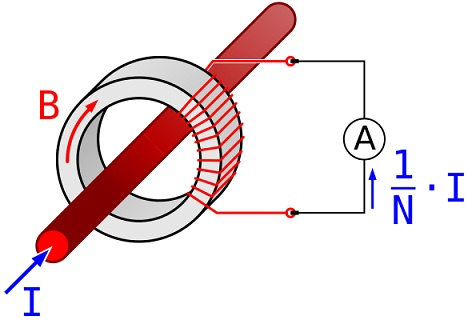# Difference Between Current Transformer and Potential Transformer

## Main Difference – Current Transformer vs. Potential Transformer

Current transformers and potential transformers are devices that allow us to measure large currents and voltages without causing damages to the measuring devices. The main difference between current transformer and potential transformer is that the current transformers use a step-up transformer in order to reduce current through its secondary coils whereas the potential transformers use a step-down transformer in order to reduce the potential difference across its secondary coil.

## What is a Current Transformer

A current transformer is a device that can be used to measure large currents in a conductor. It is a transformer, where the conductor whose current is being measured acts as the transformer’s primary winding. A ring-shaped “core” goes around the conductor, and the secondary windings are made around this ring. As the current through the “primary” conductor changes, the magnetic field induced on the ring changes. This causes the magnetic flux to change across the secondary coil, which induces a current on the secondary coil.

For a transformer, the currents in the primary and secondary coils ($I_P$ and$I_S$) and the number of windings in primary and secondary coils ($N_S$ and$N_P$) are related by:$\frac{N_P}{N_S}=\frac{I_S}{I_P}$

This means that by increasing the number of windings in the secondary coil, the current in the secondary coil could be made lower than the current in the primary coil. Since the number of “primary windings” is essentially 1 and the secondary coil has many windings, the current through the secondary coil is much lower. However, the current through the secondary coil is proportional to the current through the primary conductor. For this reason, current transformers can be used to measure currents in conductors carrying large currents without causing damage to the measuring device.Current transformers used to measure current in the wires.

Clamp meters” which are used to measure currents are also a type of current transformer. Current transformers are series transformers, which are connected in series with the conductor.

## What is a Potential Transformer

Potential transformers allow voltages to be measured across power supplies generating very large potential differences. They do this by using a step-down transformer. The potential difference across the in the primary and secondary coils ($V_P$ and$V_S$) and the number of windings in primary and secondary coils ($N_S$ and$N_P$) are related by:$\frac{N_P}{N_S}=\frac{V_P}{V_S}$

Here, a smaller potential difference can be generated across the secondary coil by making the number of windings in the secondary coil small than the number of windings across the primary coil. The potential difference across the secondary coil is proportional to the potential difference across the primary coil. Therefore, a voltmeter can be then connected across the secondary coil to measure the voltage across the primary coil, without causing any damage to the voltmeter. Note that potential transformers are parallel transformers.A potential transformer

## Difference Between Current Transformer and Potential Transformer

### Use:

Current transformers enable us to measure very large currents by producing a relatively small current in a transformer.

Voltage transformers enable us to measure large potential differences by producing a relatively small potential difference.

### Secondary Coil:

Current transformers need to have a large number of windings in its secondary coil.

Voltage transformers need to have a small number of windings in the secondary coil.

Series vs Parallel:

Current transformers are series transformers.

Voltage transformers are parallel transformers.

Step-up vs Step-down:

Current transformers are step-up transformers.

Voltage transformers are step-down transformers.

Image Courtesy:

“de:Stromwandler” by Biezl (Own work) [CC BY-SA 3.0], via Wikimedia Commons

“Current transformers used as part of metering equipment for three-phase 400Amp electricity supply…” [CC BY-SA 2.5], via Wikimedia Commons